GeeksforGeeks App
Open AppBrowser
Continue

# Introduction to Amortized Analysis

Amortized Analysis is used for algorithms where an occasional operation is very slow, but most of the other operations are faster. In Amortized Analysis, we analyze a sequence of operations and guarantee a worst-case average time that is lower than the worst-case time of a particularly expensive operation.
The example data structures whose operations are analyzed using Amortized Analysis are Hash Tables, Disjoint Sets, and Splay Trees.

Amortized analysis is a technique used in computer science to analyze the average-case time complexity of algorithms that perform a sequence of operations, where some operations may be more expensive than others. The idea is to spread the cost of these expensive operations over multiple operations, so that the average cost of each operation is constant or less.

For example, consider the dynamic array data structure that can grow or shrink dynamically as elements are added or removed. The cost of growing the array is proportional to the size of the array, which can be expensive. However, if we amortize the cost of growing the array over several insertions, the average cost of each insertion becomes constant or less.

Amortized analysis provides a useful way to analyze algorithms that perform a sequence of operations where some operations are more expensive than others, as it provides a guaranteed upper bound on the average time complexity of each operation, rather than the worst-case time complexity.

Amortized analysis is a method used in computer science to analyze the average performance of an algorithm over multiple operations. Instead of analyzing the worst-case time complexity of an algorithm, which gives an upper bound on the running time of a single operation, amortized analysis provides an average-case analysis of the algorithm by considering the cost of several operations performed over time.

The key idea behind amortized analysis is to spread the cost of an expensive operation over several operations. For example, consider a dynamic array data structure that is resized when it runs out of space. The cost of resizing the array is expensive, but it can be amortized over several insertions into the array, so that the average time complexity of an insertion operation is constant.

Amortized analysis is useful for designing efficient algorithms for data structures such as dynamic arrays, priority queues, and disjoint-set data structures. It provides a guarantee that the average-case time complexity of an operation is constant, even if some operations may be expensive.

Let us consider an example of simple hash table insertions. How do we decide on table size? There is a trade-off between space and time, if we make hash-table size big, search time becomes low, but the space required becomes high.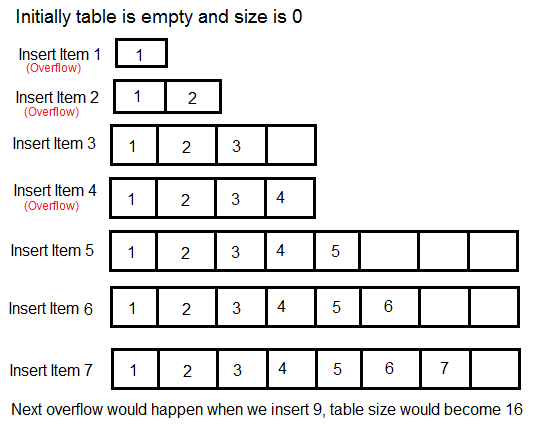The solution to this trade-off problem is to use Dynamic Table (or Arrays). The idea is to increase the size of the table whenever it becomes full. Following are the steps to follow when the table becomes full.
1) Allocate memory for larger table size, typically twice the old table.
2) Copy the contents of the old table to a new table.
3) Free the old table.

If the table has space available, we simply insert a new item in the available space.

What is the time complexity of n insertions using the above scheme?
If we use simple analysis, the worst-case cost of insertion is O(n). Therefore, the worst-case cost of n inserts is n * O(n) which is O(n2). This analysis gives an upper bound, but not a tight upper bound for n insertions as all insertions don’t take Θ(n) time.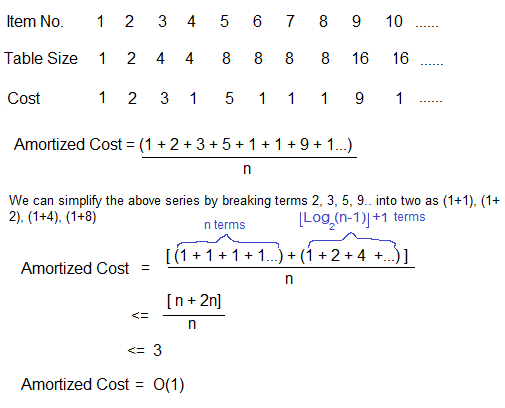So using Amortized Analysis, we could prove that the Dynamic Table scheme has O(1) insertion time which is a great result used in hashing. Also, the concept of the dynamic table is used in vectors in C++ and ArrayList in Java

Following are a few important notes.
1) Amortized cost of a sequence of operations can be seen as expenses of a salaried person. The average monthly expense of the person is less than or equal to the salary, but the person can spend more money in a particular month by buying a car or something. In other months, he or she saves money for the expensive month.

2) The above Amortized Analysis was done for Dynamic Array example is called Aggregate Method. There are two more powerful ways to do Amortized analysis called Accounting Method and Potential Method. We will be discussing the other two methods in separate posts.

3) The amortized analysis doesn’t involve probability. There is also another different notion of average-case running time where algorithms use randomization to make them faster and the expected running time is faster than the worst-case running time. These algorithms are analyzed using Randomized Analysis. Examples of these algorithms are Randomized Quick Sort, Quick Select and Hashing. We will soon be covering Randomized analysis in a different post.

Amortized analysis of insertion in Red-Black Tree

Let us discuss the Amortized Analysis of Red-Black Tree operations (Insertion) using the Potential Method.

To perform the amortized analysis of the Red-Black Tree Insertion operation, we use the Potential(or Physicist’s) method. For the potential method, we define a potential functionthat maps a data structure to a non-negative real value. An operation can result in a change of this potential.

Let us define the potential functionin the following manner:

(1)where n is a node of Red-Black Tree

Potential function,over all nodes of the red black tree.

Further, we define the amortized time of an operation as:

Amortized time= c +(h)(h)=(h’) –(h)
where h and h’ are the states of the Red-Black Tree before and after the operation respectively
c is the actual cost of the operation

The change in potential should be positive for low-cost operations and negative for high-cost operations.

A new node is inserted on a leaf of a red-black tree. We have the leaves of a red-black tree of any one of the following types: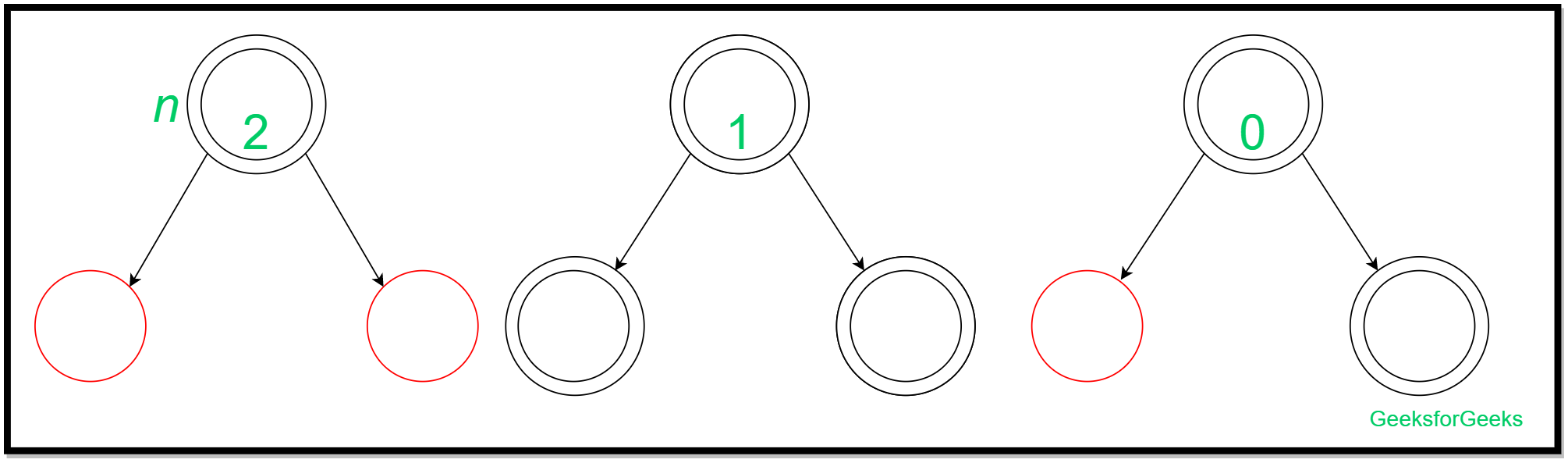The insertions and their amortized analysis can be represented as:
(1)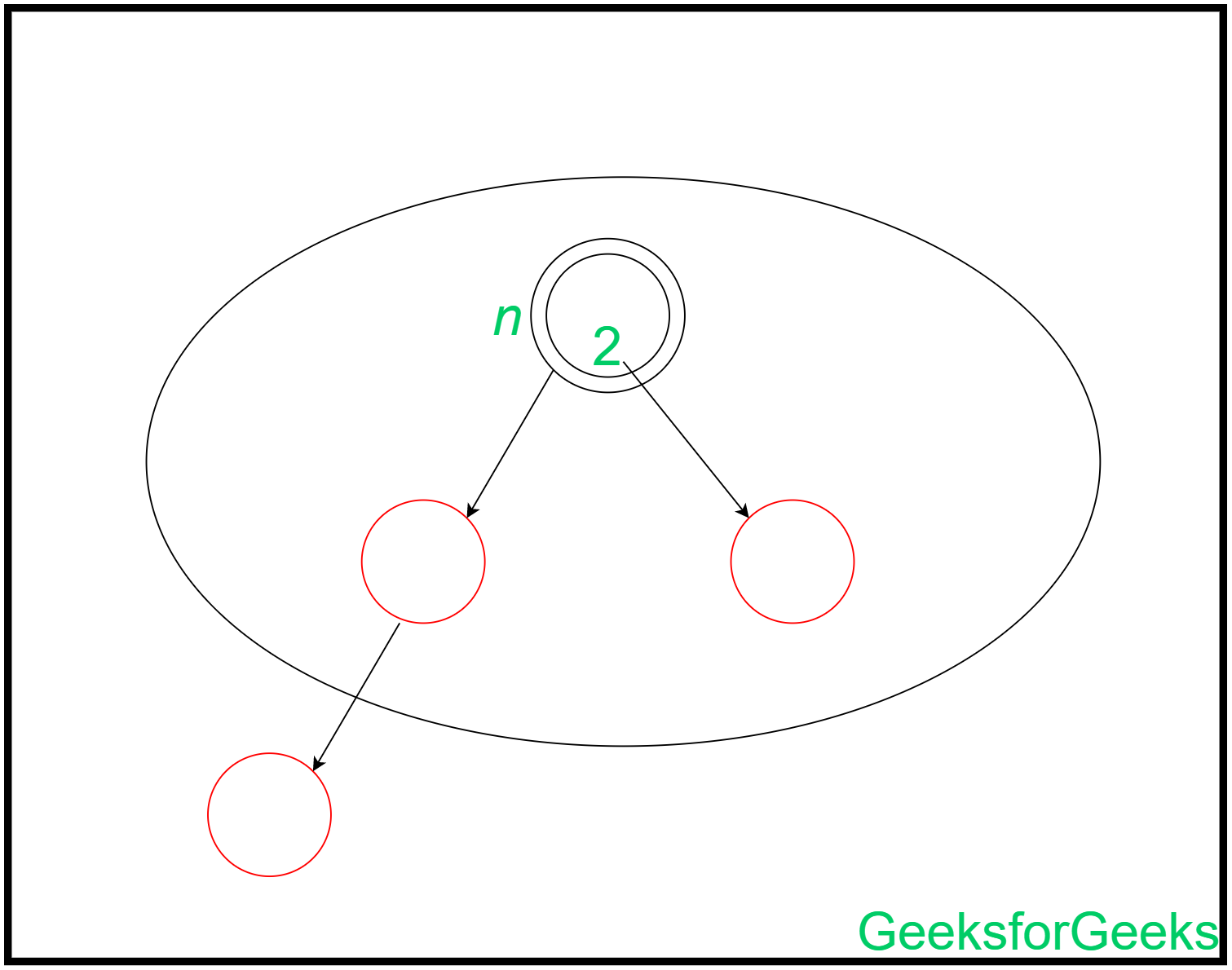This insertion is performed by first recoloring the parent and the other sibling(red). Then the grandparent and uncle of that leaf node are considered for further recoloring which leads to the amortized cost to be -1(when the grandparent of the leaf node is red), -2 (when uncle of the leaf is black and the grandparent is black) or +1 (when uncle of the leaf is red and grandparent is black). The insertion can be shown as: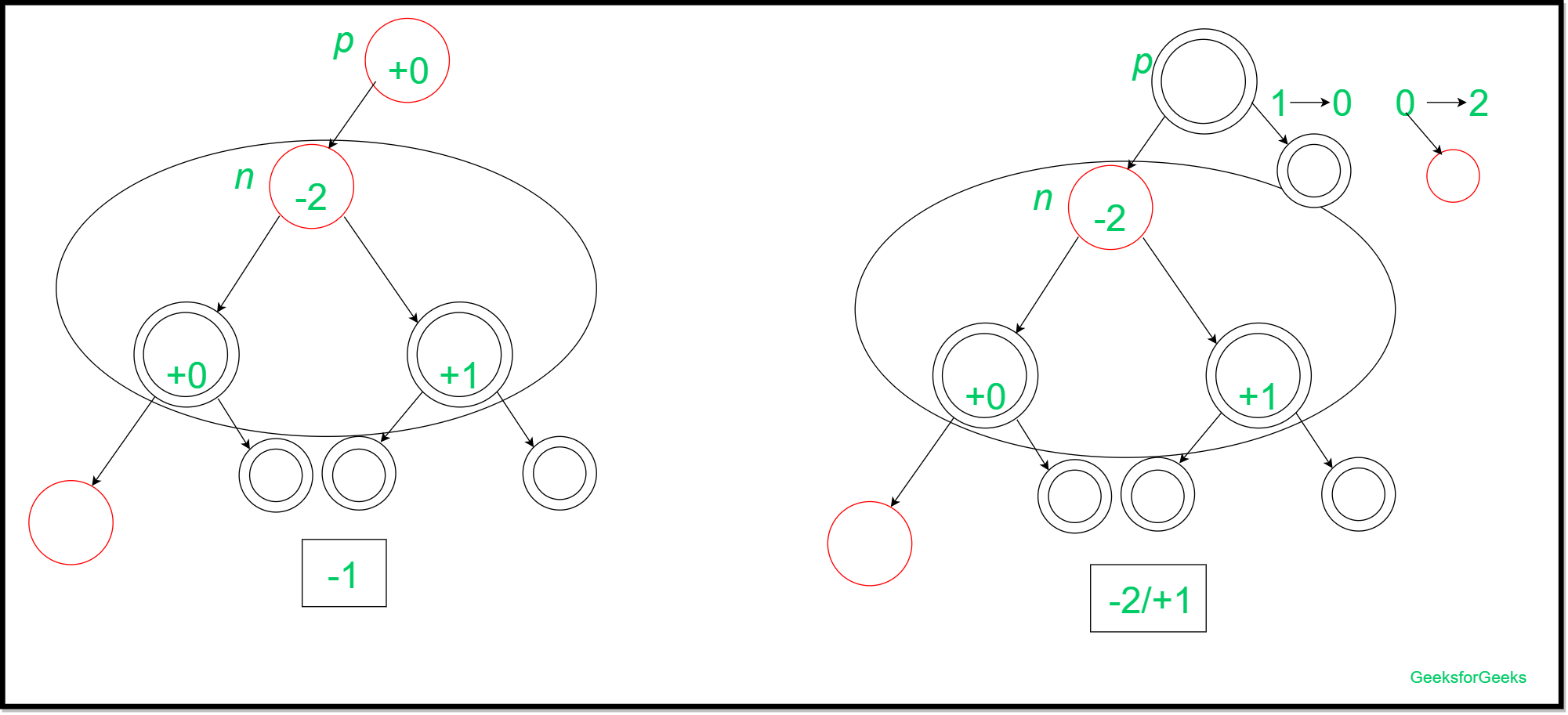(2)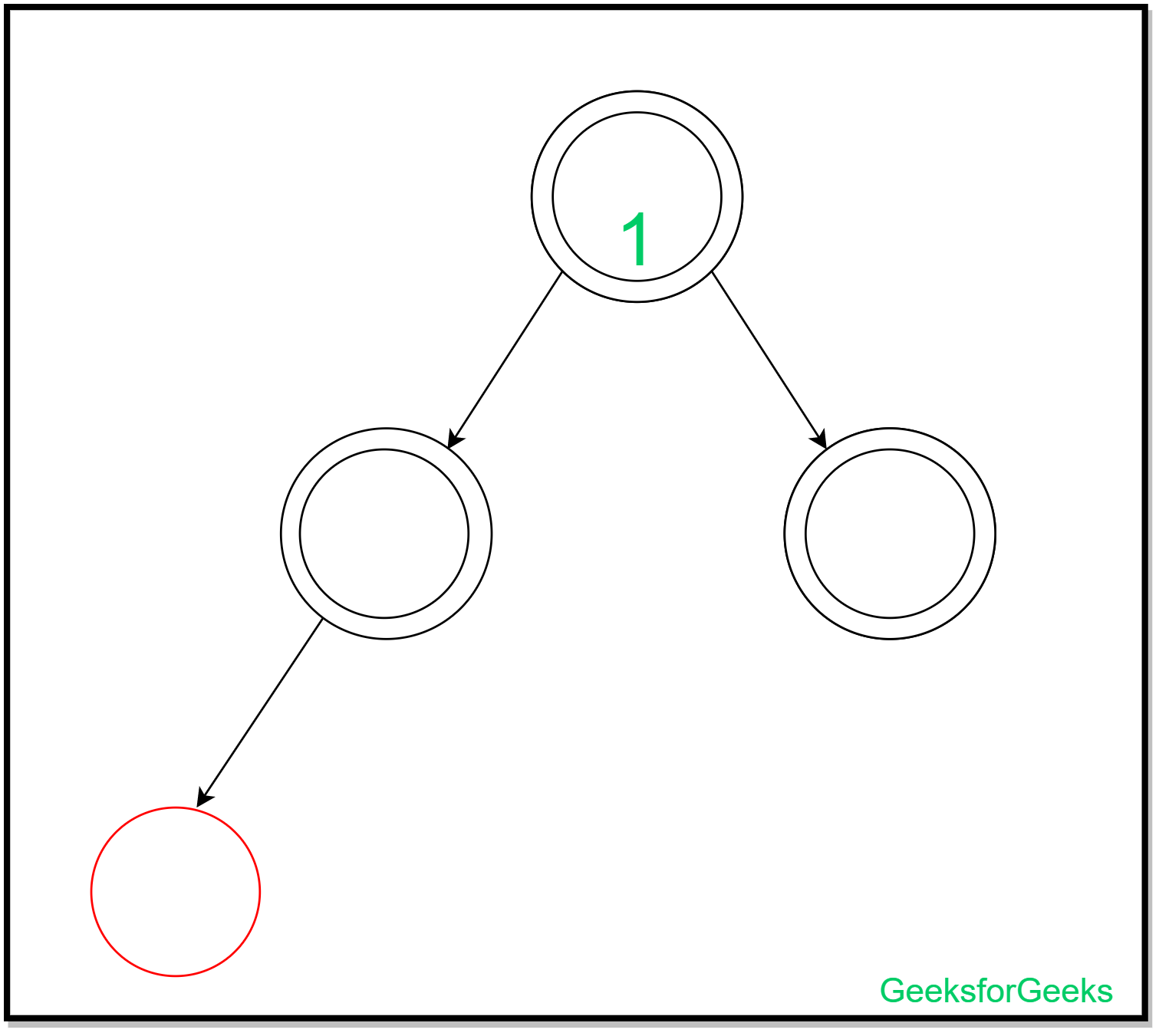In this insertion, the node is inserted without any changes as the black depth of the leaves remains the same. This is the case when the leaf may have a black sibling or does not have any sibling (since we consider the color of the color of null node to be black).

So, the amortized cost of this insertion is 0

(3)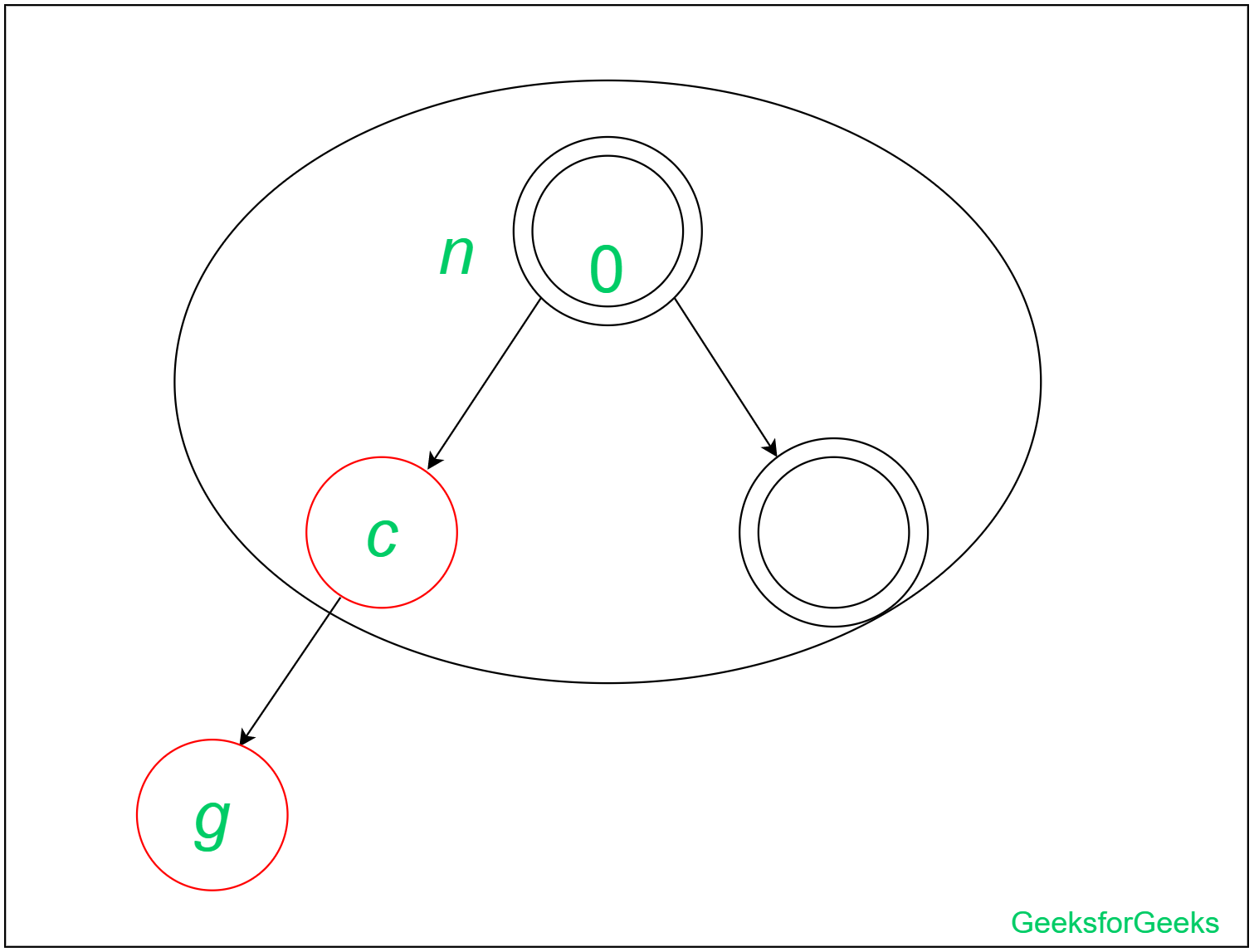In this insertion, we cannot recolor the leaf node, its parent, and the sibling such that the black depth stays the same as before. So, we need to perform a Left- Left rotation.

After rotation, there are no changes when the grandparent of g(the inserted node) is black. Also, for the case of the Red Grandparent of g(the inserted node), we do not have any changes. So, the insertion is completed with amortized cost= +2. The insertion has been depicted below: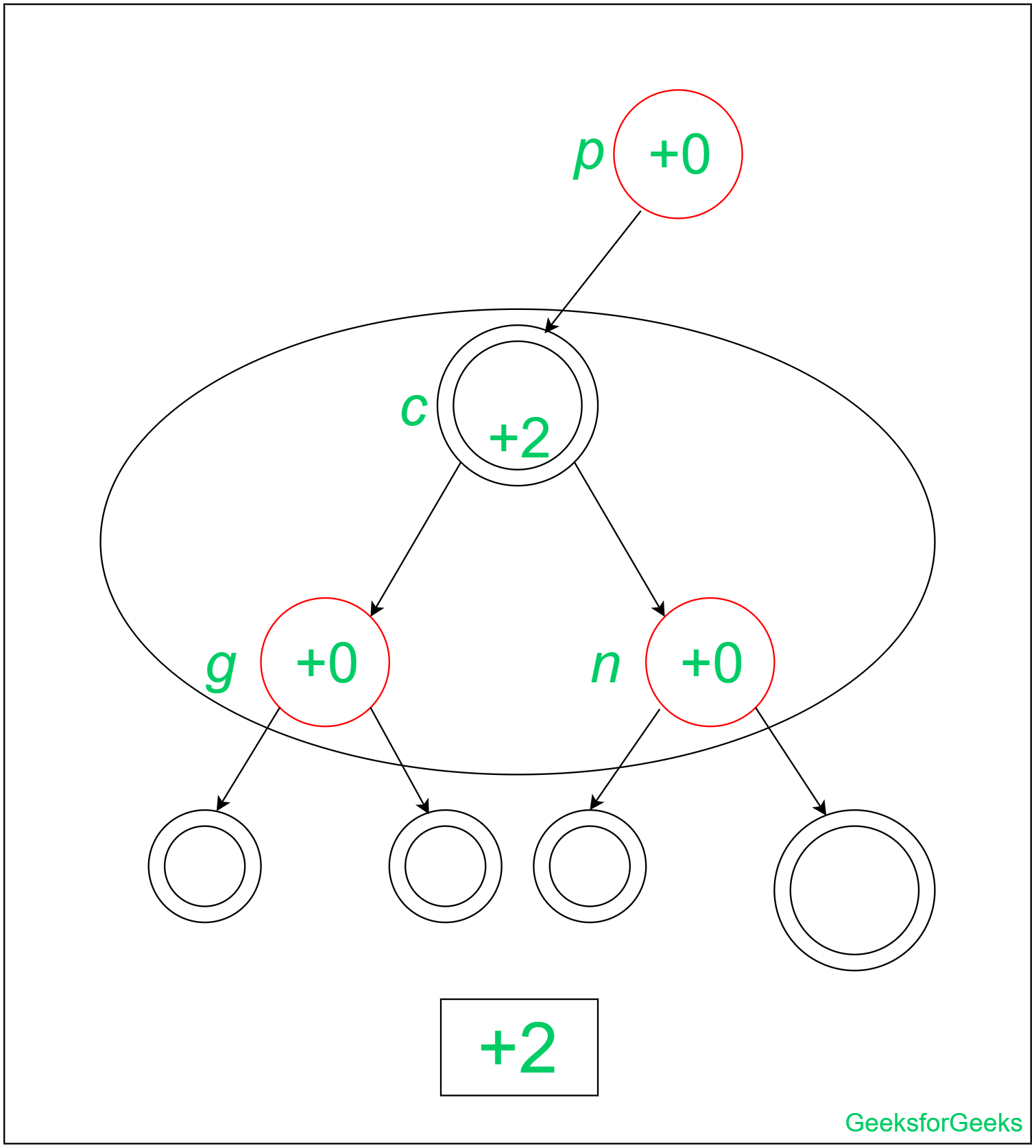After calculating these particular amortized costs at the leaf site of a red-black tree we can discuss the nature of the total amortized cost of insertion in a red-black tree. Since this may happen that two red nodes may have a parent-child relationship till the root of the red-black tree.

So in the extreme(or corner) case, we reduce the number of black nodes with two red children by 1, and we at most increase the number of black nodes with no red children by 1, leaving a net loss of at most 1 to the potential function. Since one unit of potential pays for each operation therefore(h)where n is the total number of nodes

In computer science and algorithms, amortized analysis is a technique used to estimate the average time complexity of an algorithm over a sequence of operations, rather than the worst-case complexity of individual operations. It allows us to make more accurate predictions about the overall efficiency of an algorithm, especially in cases where some operations may take longer than others.

1. The basic idea behind amortized analysis is to distribute the cost of expensive operations over a sequence of less expensive operations. For example, suppose we have an algorithm that occasionally performs a very expensive operation that takes O(n) time, but most of the time performs an operation that takes only O(1) time. In worst-case analysis, we would say that the algorithm takes O(n) time, but in amortized analysis, we can distribute the cost of the expensive operation over a sequence of n operations, resulting in an average cost of O(1) per operation.
2. There are several techniques for performing amortized analysis, including aggregate analysis, accounting method, and potential method. In aggregate analysis, we compute the total cost of a sequence of operations and divide it by the number of operations to get the average cost per operation. In the accounting method, we assign credits to each operation and use them to pay for expensive operations. In the potential method, we assign a potential value to the data structure being operated on and use it to measure the amount of work done by each operation.

Amortized analysis is a powerful tool for analyzing the performance of algorithms over a sequence of operations. It allows us to make more accurate predictions about the average case complexity of an algorithm and can help us identify cases where an algorithm may perform poorly in practice, even if its worst-case complexity is low.

1. More accurate predictions: Amortized analysis provides a more accurate prediction of the average-case complexity of an algorithm over a sequence of operations, rather than just the worst-case complexity of individual operations.
2. Provides insight into algorithm behavior: By analyzing the amortized cost of an algorithm, we can gain insight into how it behaves over a longer period of time and how it handles different types of inputs.
3. Helps in algorithm design: Amortized analysis can be used as a tool for designing algorithms that are efficient over a sequence of operations.
Useful in dynamic data structures: Amortized analysis is particularly useful in dynamic data structures like heaps, stacks, and queues, where the cost of an operation may depend on the current state of the data structure.

1. Complexity: Amortized analysis can be complex, especially when multiple operations are involved, making it difficult to implement and understand.
2. Limited applicability: Amortized analysis may not be suitable for all types of algorithms, especially those with highly unpredictable behavior or those that depend on external factors like network latency or I/O operations.
3. Lack of precision: Although amortized analysis provides a more accurate prediction of average-case complexity than worst-case analysis, it may not always provide a precise estimate of the actual performance of an algorithm, especially in cases where there is high variance in the cost of operations.

Thus, the total amortized cost of insertion in the Red-Black Tree is O(n)

For any doubts regarding insertions in a red-black tree, you may refer to Insertions in Red-Black Tree

For more details, please refer: Design and Analysis of Algorithms.